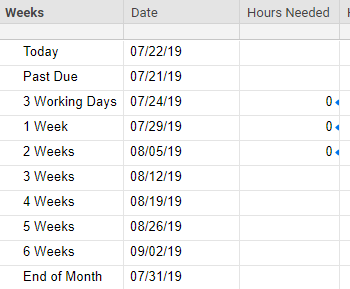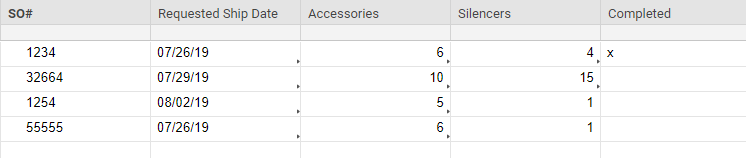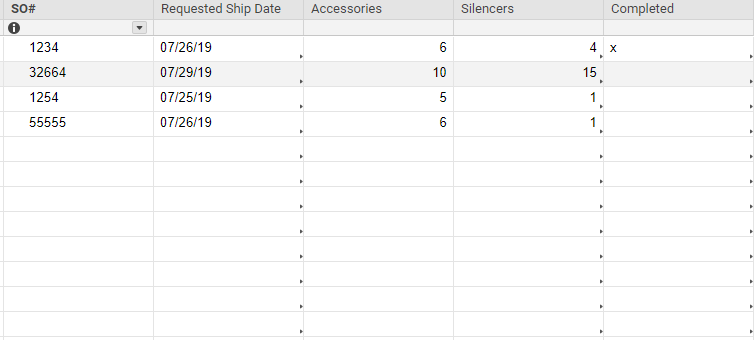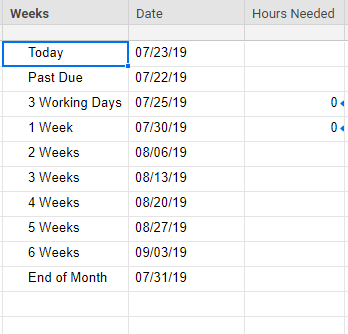# SUMIFS, cross-sheet reference based on dates.

Sorry if this is a duplicate. I couldn't find an answer that fit my problem..

Formula: =SUMIFS({AccHours}, {ShipDate}, <=Date3, {completed}, <>"x")

All references are from another sheet except Date3. Date3 is a formula as well, which is TODAY()+2. This works in Excel, but doesn't seem to work here. I don't get an error, but instead I get zero. I still get zero even if I change the Date3 reference to a date instead of a formula.• What do you have in rows 4 and 5? Based on your screenshots, row 3 SHOULD be 0.

• Those rows are not used yet. The row with the latest July date should be greater than zero.

• That's what I am asking. What is the formula in the row with the latest July date?

• Oh, sorry.=SUMIFS({AccHours}, {ShipDate}, <=Date4, {completed}, <>"x")

Date4 is the July date next to the column. Does that help?

• It does. Thank you.

Now... What all is included in the {AccHours} reference?

I also see that the first two rows in the Silencers column are linked out to somewhere. Do they play into any of this at all?

• I actually named that reference wrong. It should be referencing the column Silencers on the other sheet.

The formula is supposed to do this:

Sum the hours in the silencer column if the ship date is less than or equal to the date in the left column (Date3). Then, if the Completed column has an x in it, don't count it because it's been shipped.

=SUMIFS({SilHours}, {ShipDate}, <=Date3, {completed}, <>"x")

• Ok. I am going to suggest that you start by double checking the ranges. You are wanting to reference the entire Silencers column, but only the first two rows are linked as opposed to the entire column.

It may be that one or more ranges are off.

I am also curious to see the far right side of the Completed column. It doesn't look like that column is linked out to anywhere, but it could be that your screenshot just trimmed that edge of the cell off.

• I changed the reference to the entire columns for each item, but it still didn't work. I've attached updated screenshots.

Thanks so much for taking the time to look at this!• How are each of the dates populated and what column types are they in?

How are your totals populated that you are wanting to sum and what type of column are they in?

• EDIT: I took out the reference to the "x" and it worked. Looks like I have a problem with that reference.

The dates are formulas. Today's is using TODAY(). The one below is using that result + 1. However, I've tried changing them to solid dates instead of a formula and that didn't work.

Date column is date format. The totals and the hours to total are Text/Number format.

• Try using an @cell reference...

@cell <> "x")

## Help Article Resources

Want to practice working with formulas directly in Smartsheet?

Check out the Formula Handbook template!